CRR Tool
Capital Requirements Regulation (CRR)
Article 325bc

# Article 325bc - Partial expected shortfall calculations

Status
In force
Selected consolidated version from
30/09/2021
Search all articles and keywords within this legal act

Article 325bc

Partial expected shortfall calculations

1.

Institutions shall calculate all the partial expected shortfall measures referred to in Article 325bb(1) as follows:

(a)

daily calculations of the partial expected shortfall measures;

(b)

at 97,5th percentile, one tailed confidence interval;

(c)

for a given portfolio of trading book positions and non-trading book positions that are subject to foreign exchange or commodity risk, institutions shall calculate the partial expected shortfall measure at time ‘t’ in accordance with the following formula: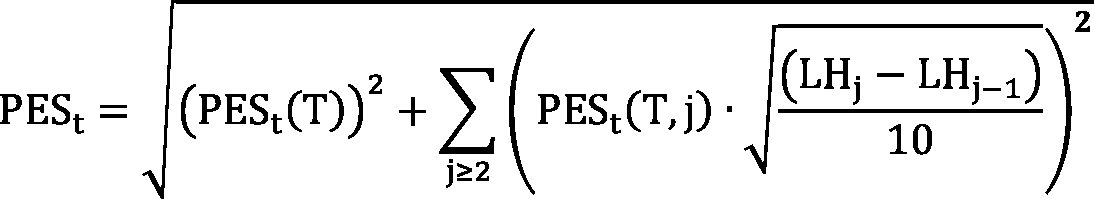where:

 PESt = the partial expected shortfall measure at time t;
 j = the index that denotes the five liquidity horizons listed in the first column of Table 1;
 LHj = the length of liquidity horizons j as expressed in days in Table 1;
 T = the base time horizon, where T = 10 days;
 PESt(T) = the partial expected shortfall measure that is determined by applying scenarios of future shocks with a 10-day time horizon only to the specific set of modellable risk factors of the positions in the portfolio set out in paragraphs 2, 3 and 4 for each partial expected shortfall measure referred to in Article 325bb(1); and

PESt(T, j)

=

the partial expected shortfall measure that is determined by applying scenarios of future shocks with a 10-day time horizon only to the specific set of modellable risk factors of the positions in the portfolio set out in paragraphs 2, 3 and 4 for each partial expected shortfall measure referred to in Article 325bb(1) and of which the effective liquidity horizon, as determined in accordance with Article 325bd(2), is equal or longer than LHj.

Table 1

 Liquidity horizon j Length of liquidity horizon j (in days) 1 10 2 20 3 40 4 60 5 120
2.
For the purpose of calculating the partial expected shortfall measures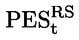and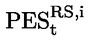referred to in Article 325bb(1), in addition to the requirements set out in paragraph 1 of this Article, institutions shall meet the following requirements:
(a)
in calculating, institutions shall only apply scenarios of future shocks to a subset of the modellable risk factors of the positions in the portfolio which has been chosen by the institution, to the satisfaction of the competent authorities, so that the following condition is met with the sum taken over from the preceding 60 business days: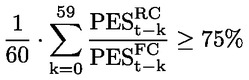An institution that no longer meets the requirement referred to in the first paragraph of this point shall immediately notify the competent authorities thereof and shall update the subset of the modellable risk factors within two weeks in order to meet that requirement; where, after two weeks, that institution has failed to meet that requirement, the institution shall revert to the approach set out in Chapter 1a to calculate the own funds requirements for market risk for some trading desks, until that institution is able to demonstrate to the competent authority that it is meeting the requirement set out in the first subparagraph of this point;

(b)
in calculating, institutions shall only apply scenarios of future shocks to the subset of the modellable risk factors of the positions in the portfolio chosen by the institution for the purposes of point (a) of this paragraph and which have been mapped to the broad risk factor category ‘i’ in accordance with Article 325bd;
(c)
the data inputs used to determine the scenarios of future shocks applied to the modellable risk factors referred to in points (a) and (b) shall be calibrated to historical data from a continuous 12-month period of financial stress that shall be identified by the institution in order to maximise the value of; for the purpose of identifying that stress period, institutions shall use an observation period starting at least from 1 January 2007, to the satisfaction of the competent authorities; and
(d)
the data inputs ofshall be calibrated to the 12-month stress period that has been identified by the institution for the purposes of point (c).
3.
For the purpose of calculating the partial expected shortfall measures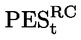and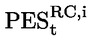referred to in Article 325bb(1), institutions shall, in addition to the requirements set out in paragraph 1 of this Article, meet the following requirements:
(a)
in calculating, institutions shall only apply scenarios of future shocks to the subset of the modellable risk factors of the positions in the portfolio referred to in point (a) of paragraph 2;
(b)
in calculating, institutions shall only apply scenarios of future shocks to the subset of the modellable risk factors of the positions in the portfolio referred to in point (b) of paragraph 2;
(c)

the data inputs used to determine the scenarios of future shocks applied to the modellable risk factors referred to in points (a) and (b) of this paragraph shall be calibrated to historical data referred to in point (c) of paragraph 4; those data shall be updated on at least a monthly basis.

4.
For the purpose of calculating the partial expected shortfall measures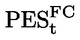and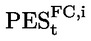referred to in Article 325bb(1), institutions shall, in addition to the requirements set out in paragraph 1 of this Article, meet the following requirements:
(a)
in calculating, institutions shall apply scenarios of future shocks to all the modellable risk factors of the positions in the portfolio;
(b)
in calculating, institutions shall apply scenarios of future shocks to all the modellable risk factors of the positions in the portfolio which have been mapped to the broad risk factor category i in accordance with Article 325bd;
(c)

the data inputs used to determine the scenarios of future shocks applied to the modellable risk factors referred to in points (a) and (b) shall be calibrated to historical data from the preceding 12-month period; where there is a significant upsurge in the price volatility of a material number of modellable risks factors of an institution's portfolio which are not in the subset of the risk factors referred to in point (a) of paragraph 2, competent authorities may require an institution to use historical data for a period shorter than the preceding 12-months, but such a shorter period shall not be shorter than the preceding six-months; competent authorities shall notify EBA of any decision to require an institution to use historical data from a shorter period than 12 months and shall substantiate that decision.

5.
In calculating a given partial expected shortfall measure as referred to in Article 325bb(1), institutions shall maintain the values of the modellable risks factors for which they have not been required to apply scenarios of future shocks for that partial expected shortfall measure under paragraphs 2, 3 and 4 of this Article.